# Frank Solutions for Class 9 Maths Chapter 15 Mid-point and Intercept Theorems

Frank Solutions for Class 9 Maths Chapter 15 Mid – point and Intercept Theorems act as an important tool in preparing for examinations. The solutions are prepared in accordance with the current ICSE board keeping in mind the understanding capacity of students. Practising solutions on a daily basis help students to achieve desired scores along with speeding up their problem solving skills. Frank Solutions are available in PDF to encourage concept based learning among students. Access Frank Solutions for Class 9 Maths Chapter 15 Mid-point and Intercept Theorems, from the available links below.

Chapter 15 has problems relying on mid-points of a triangle. Mid-point theorem of a triangle states that if the two triangle sides’ midpoints are connected then the resulting line segment is parallel to the third triangle side. Students can cross check their answers and clear doubts which appear while solving textbook problems.

## Frank Solutions for Class 9 Maths Chapter 15 Mid – point and Intercept Theorems Download PDF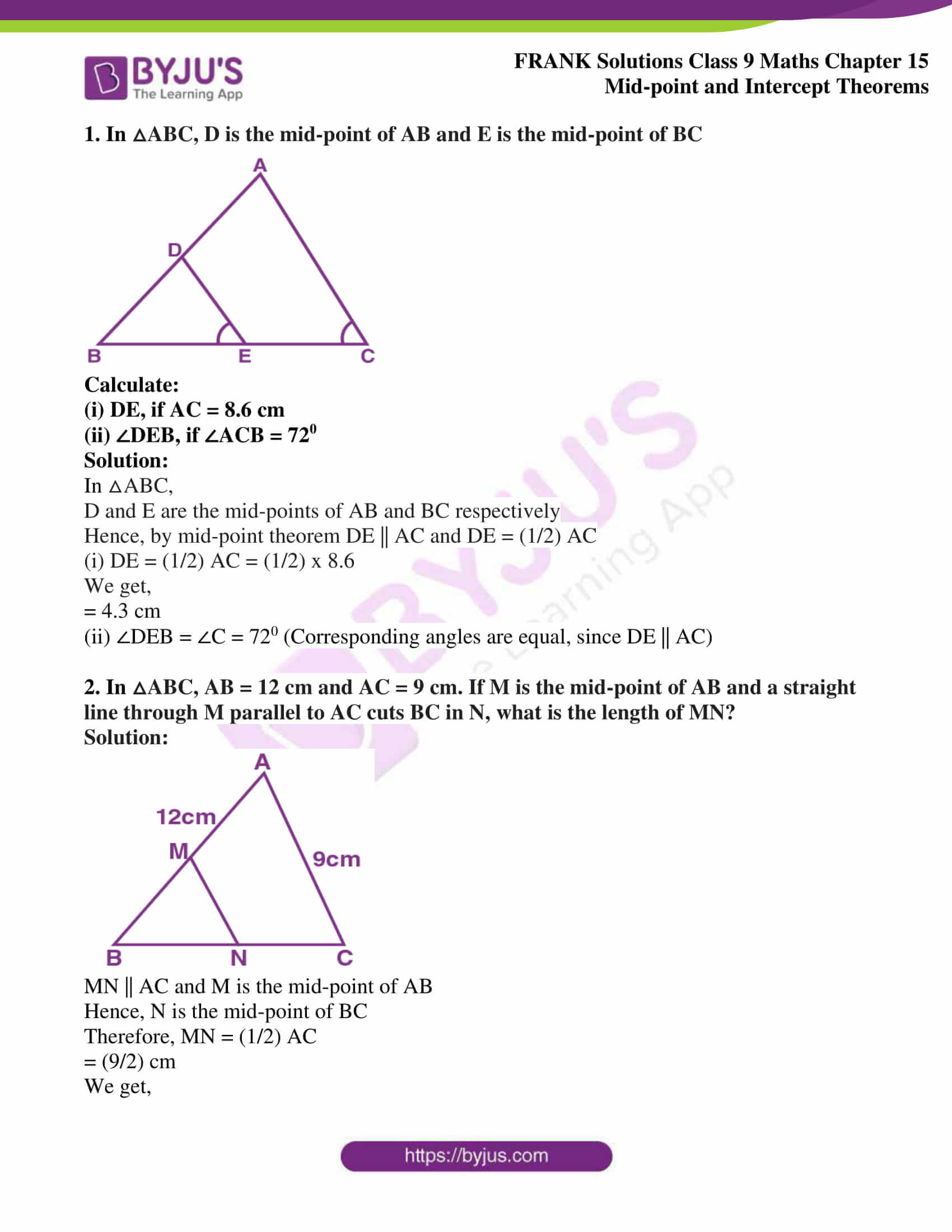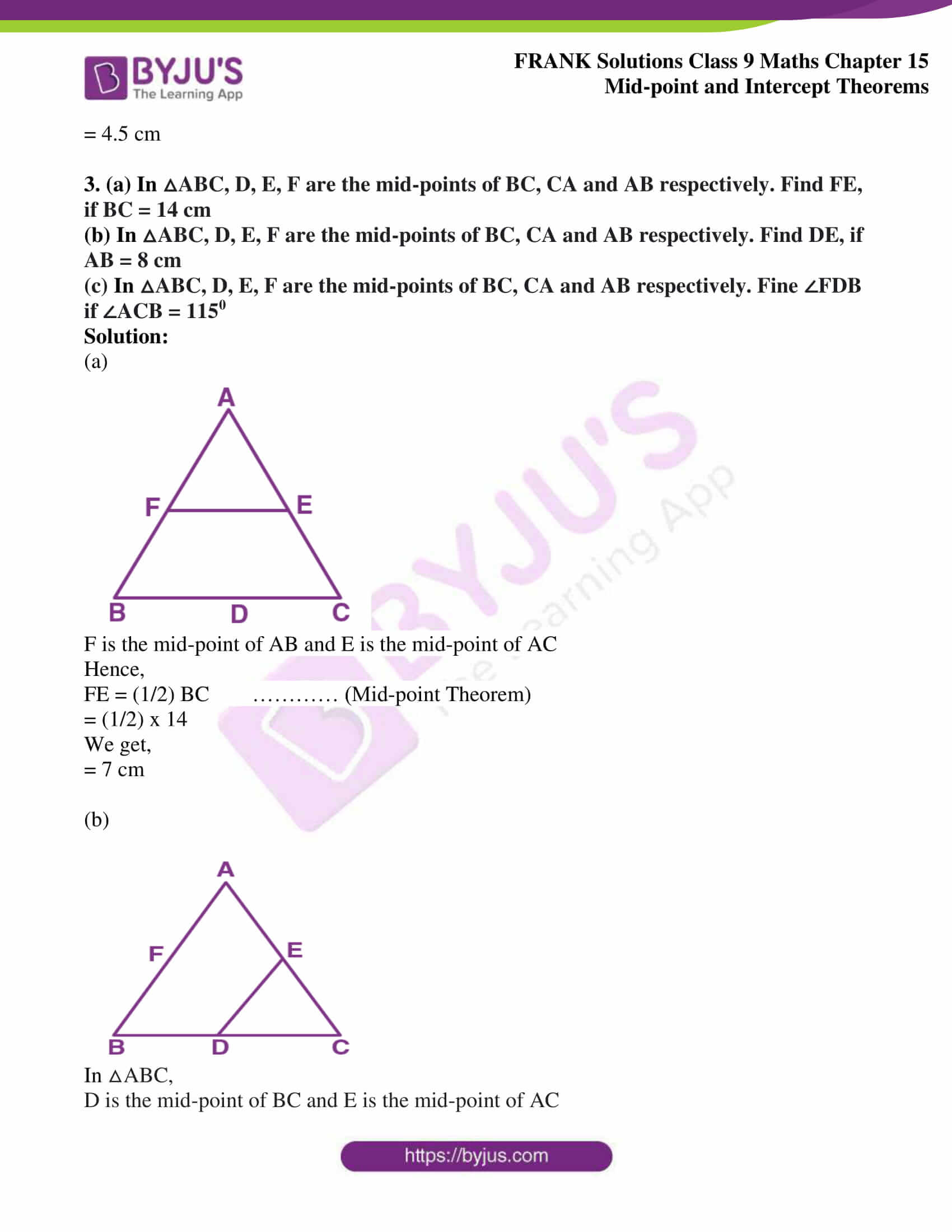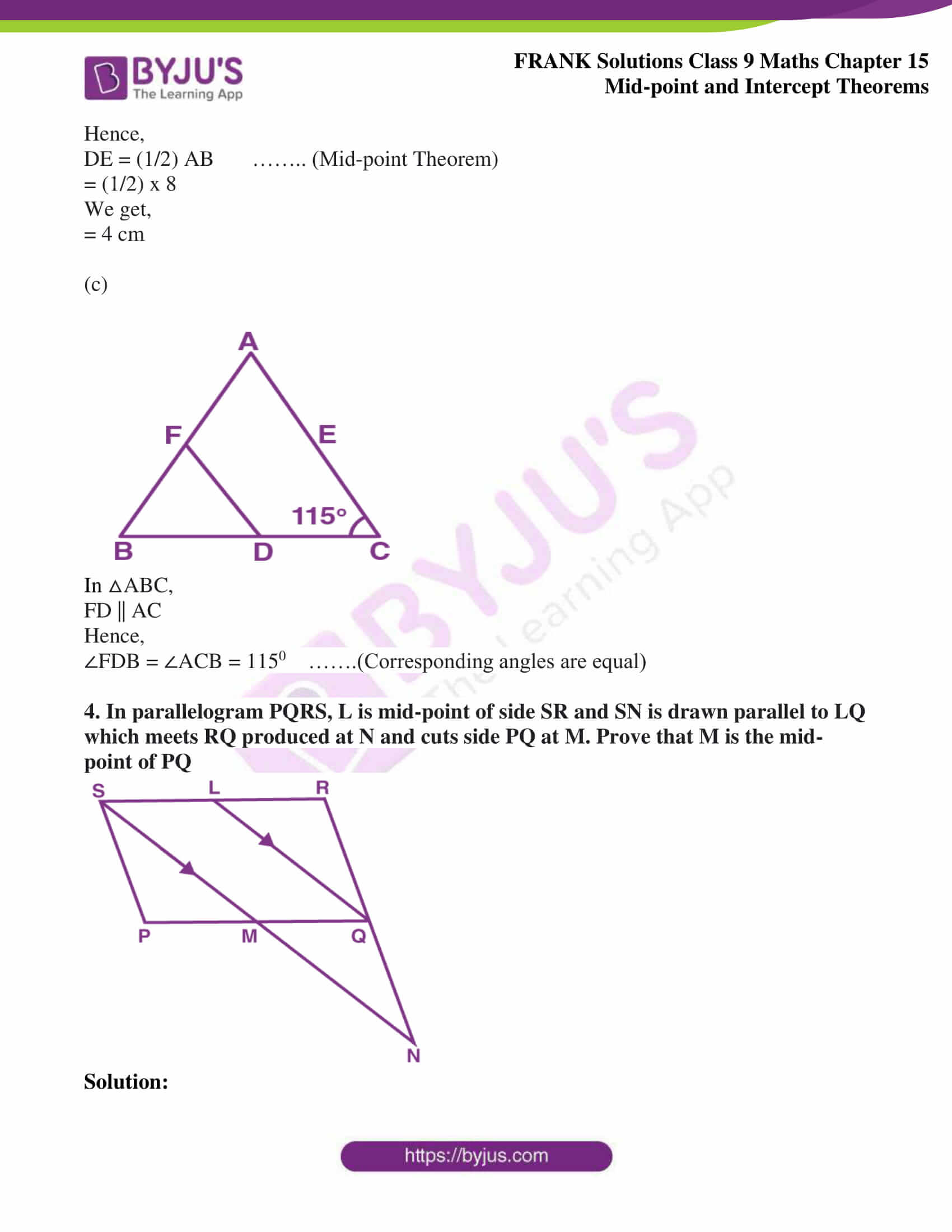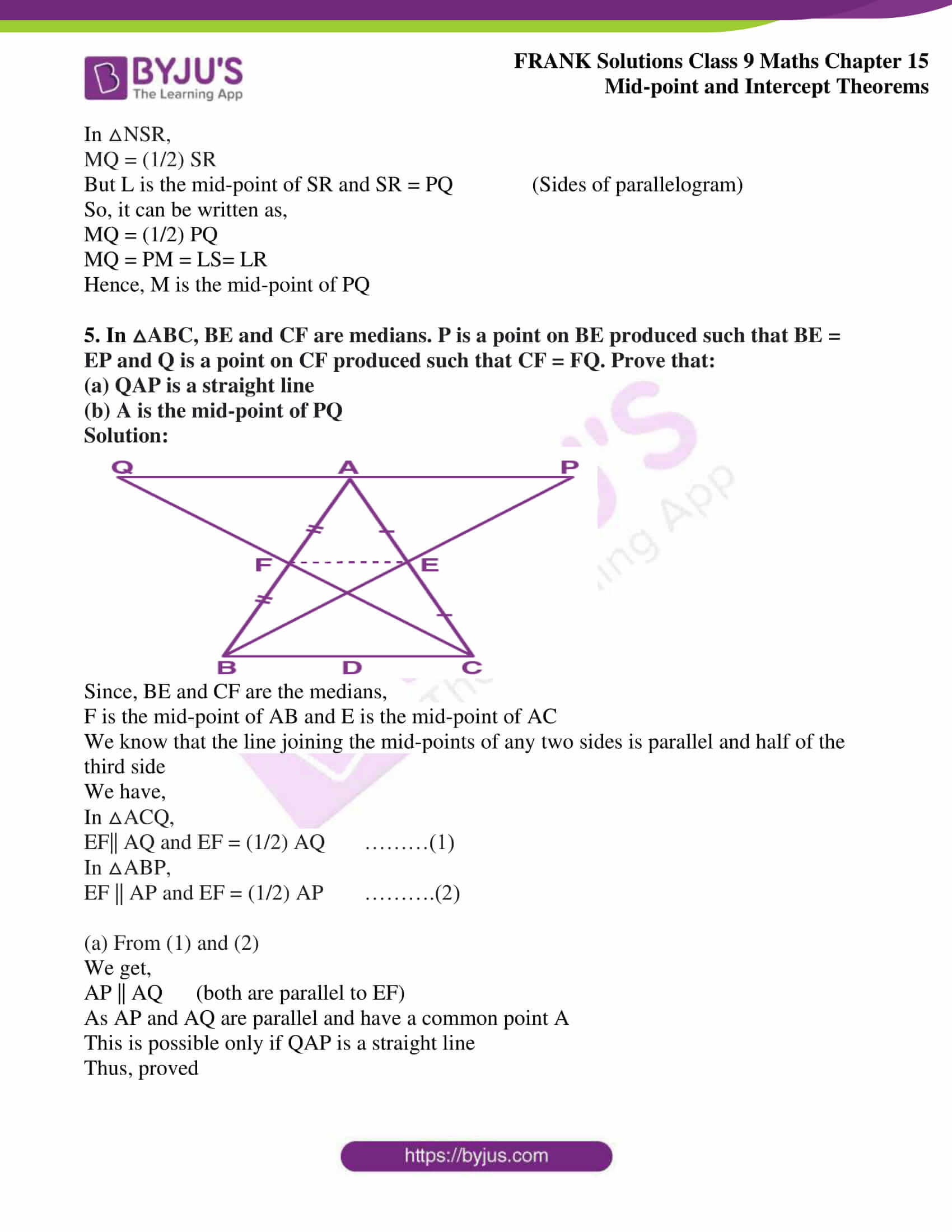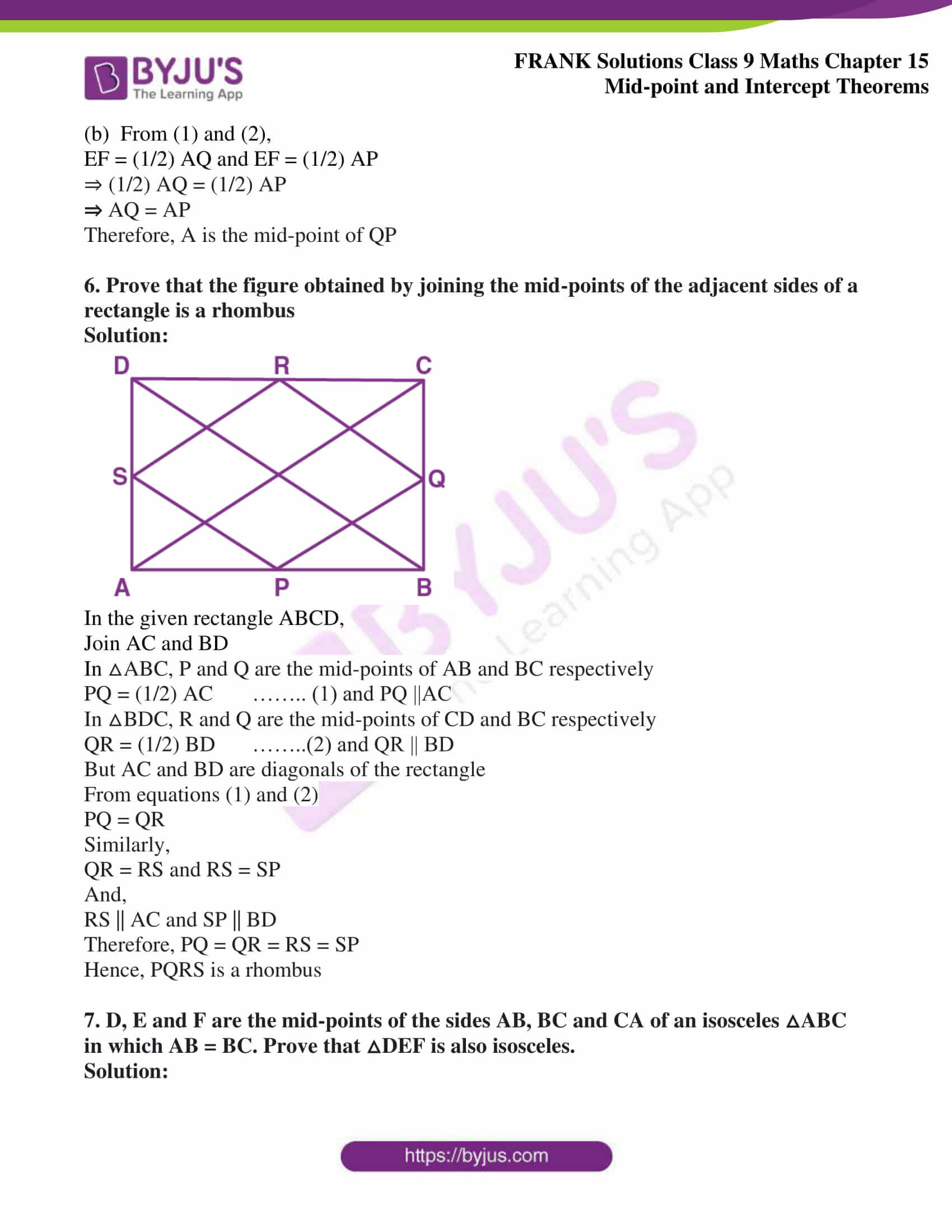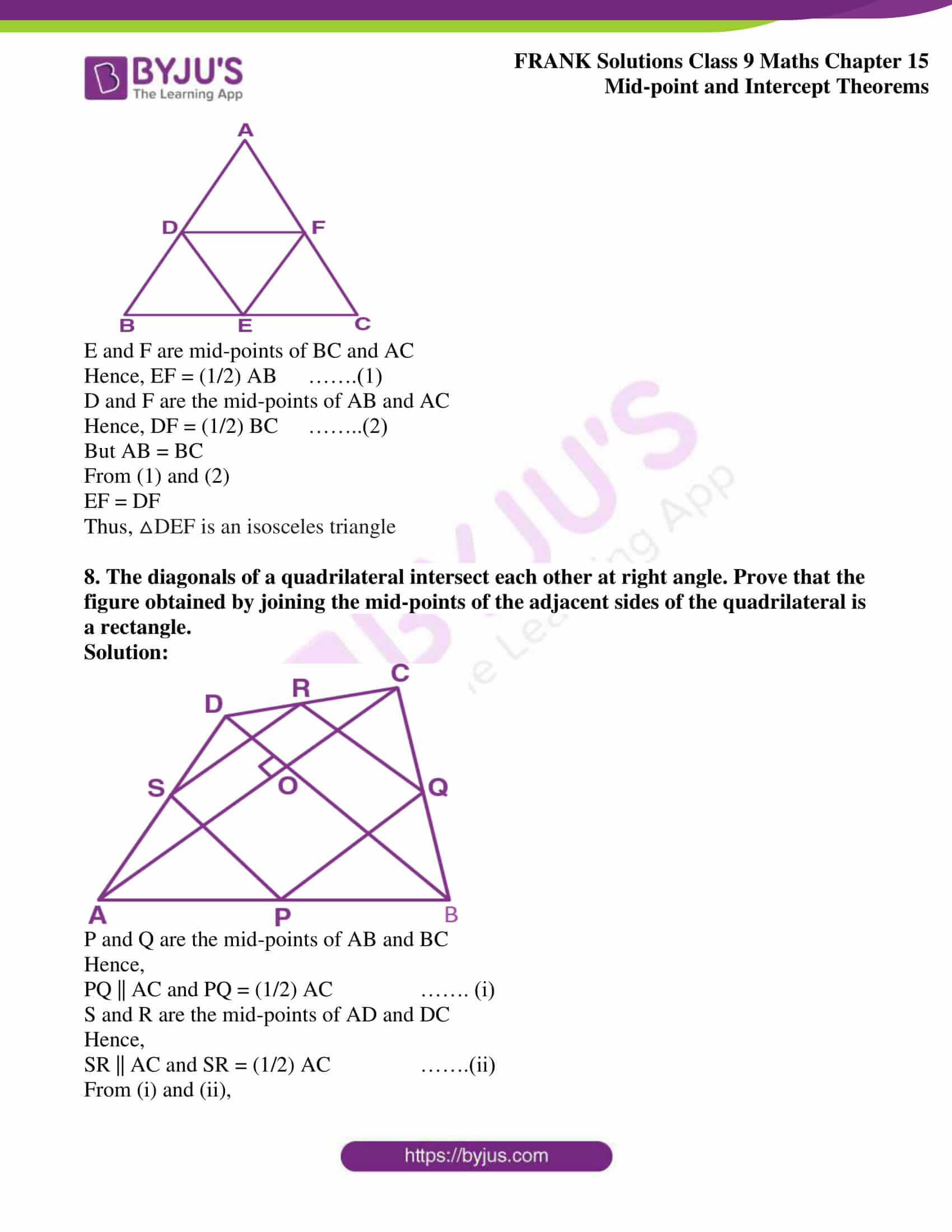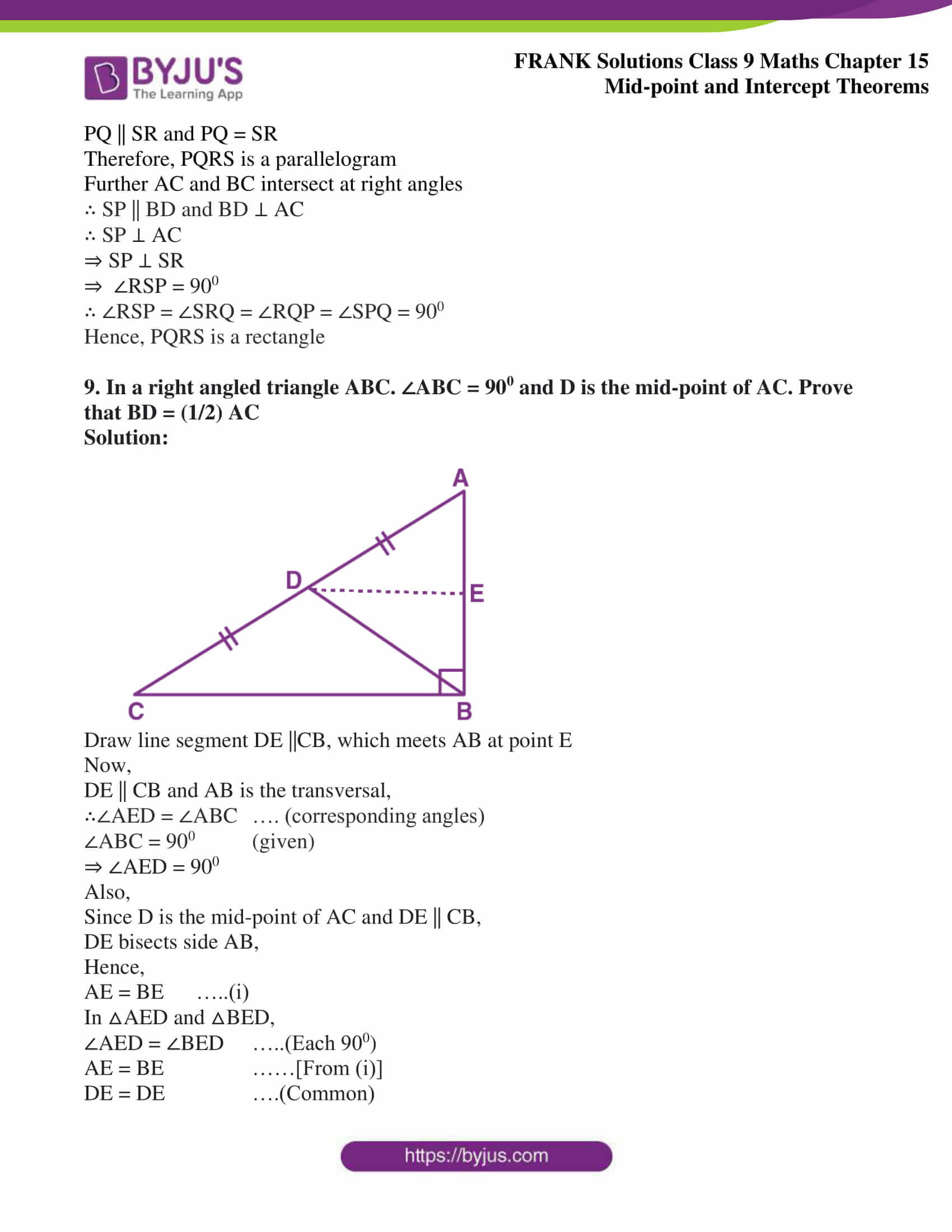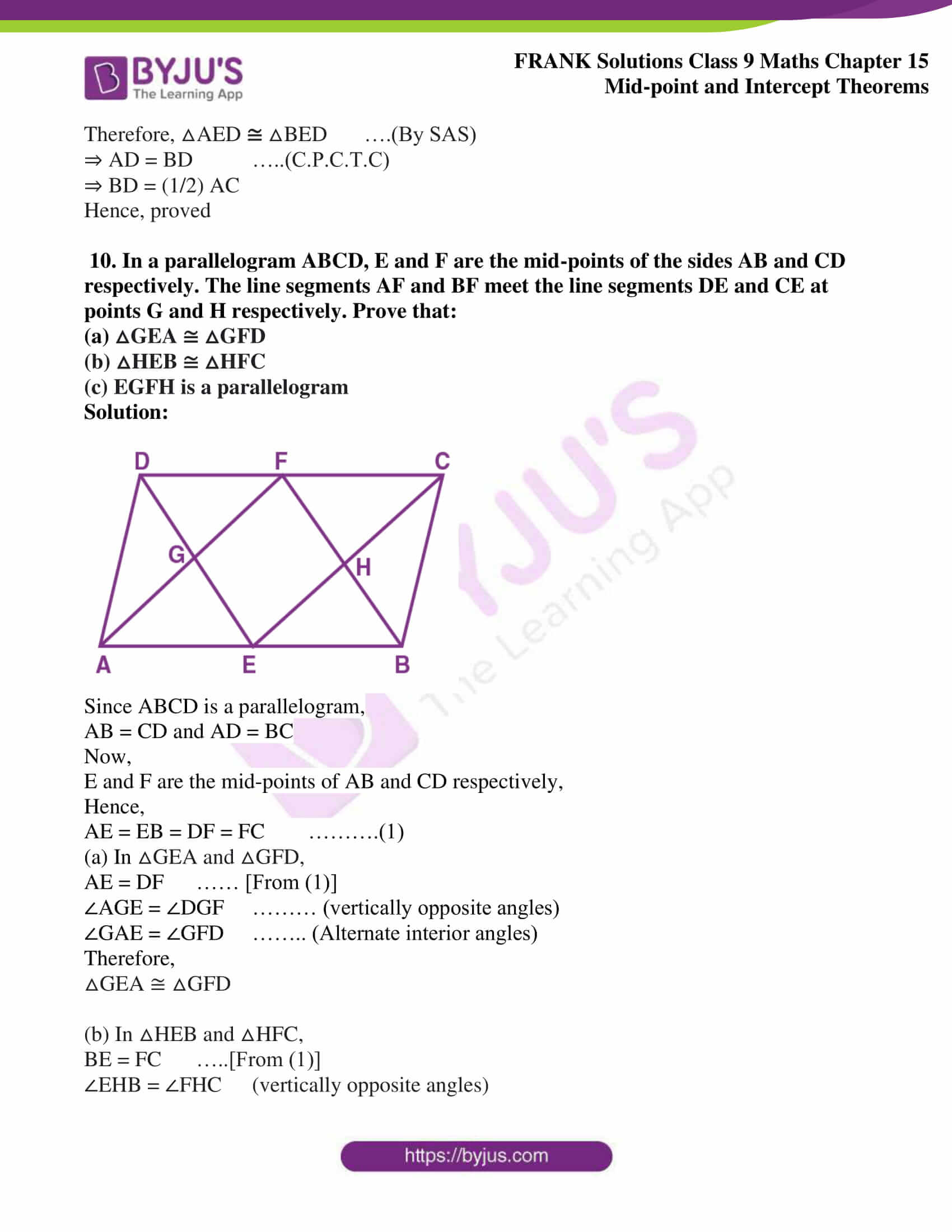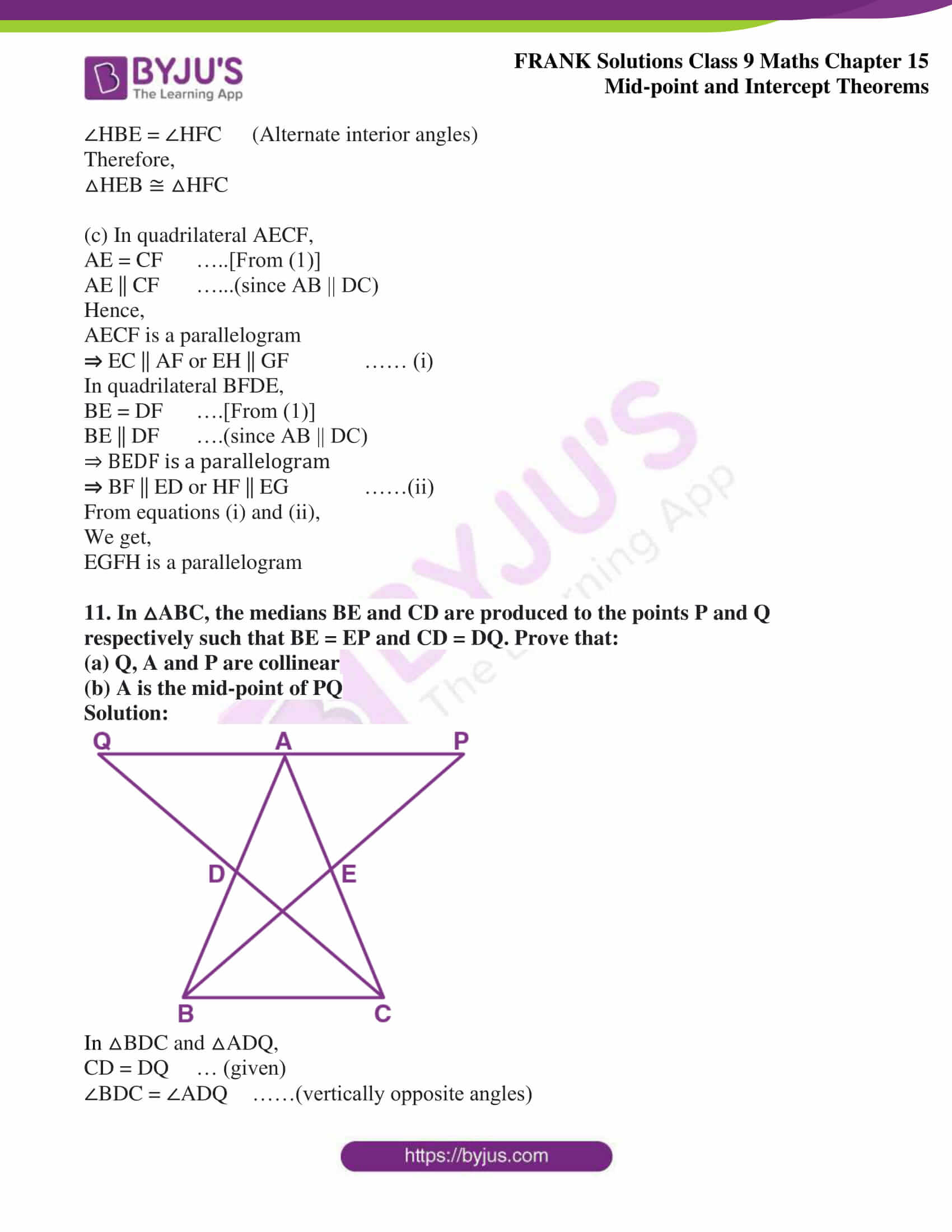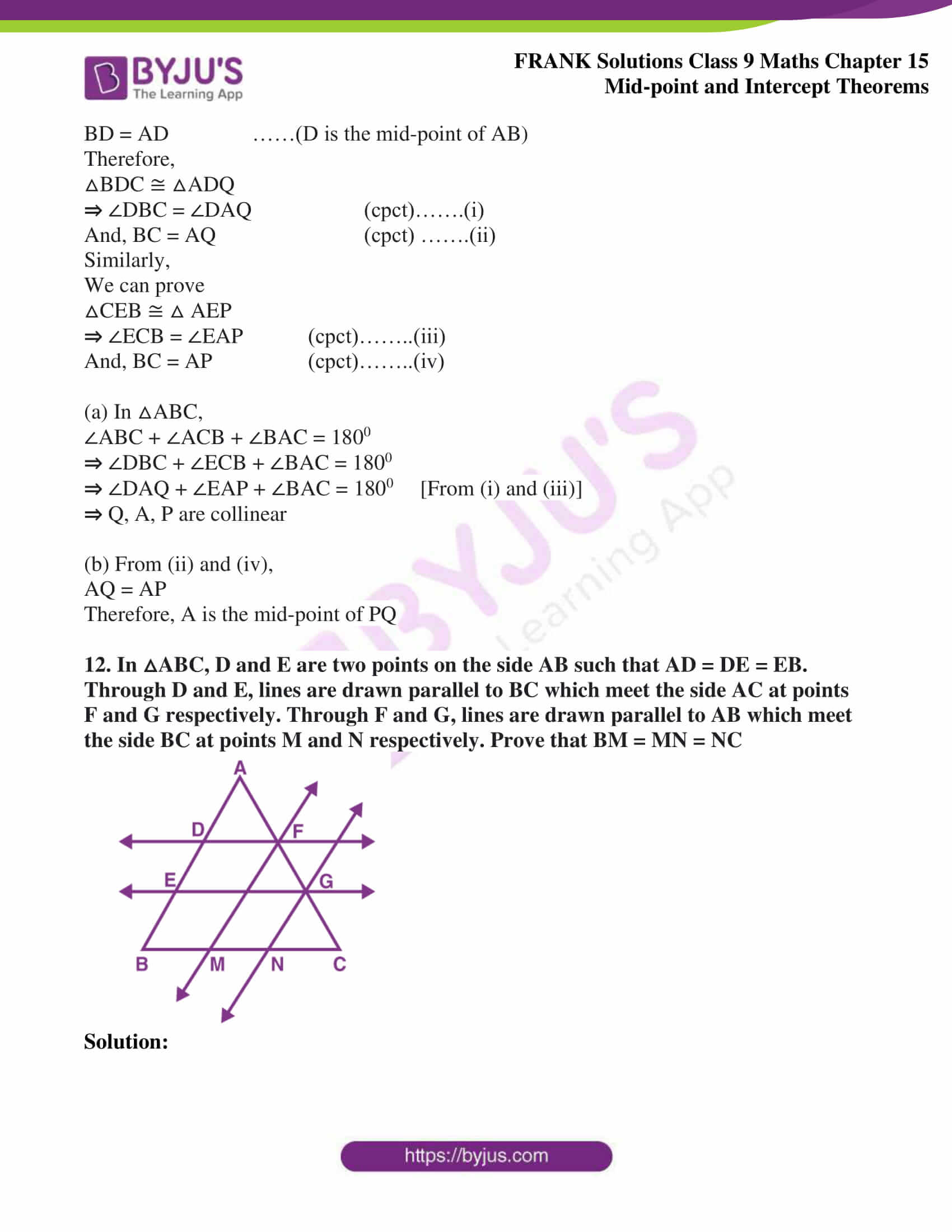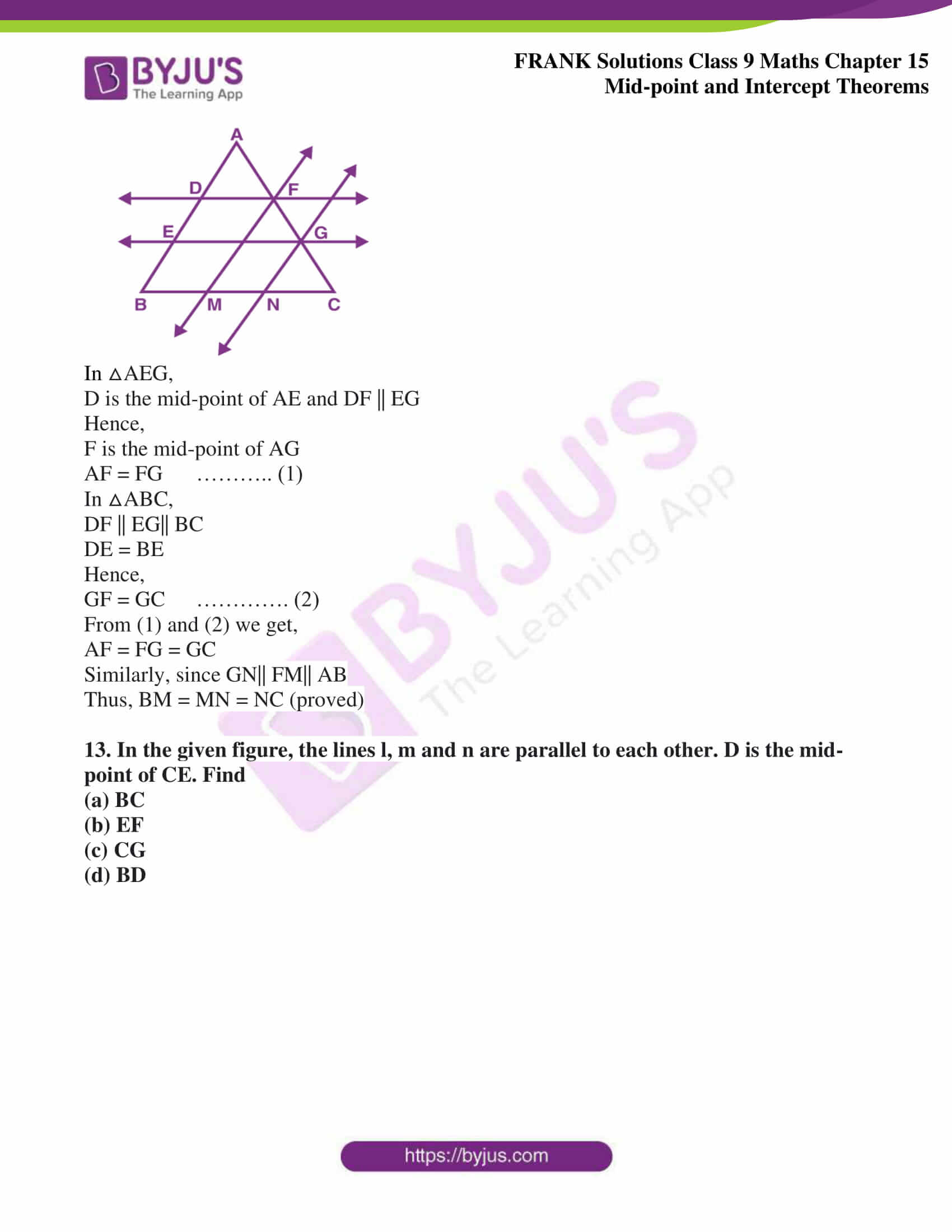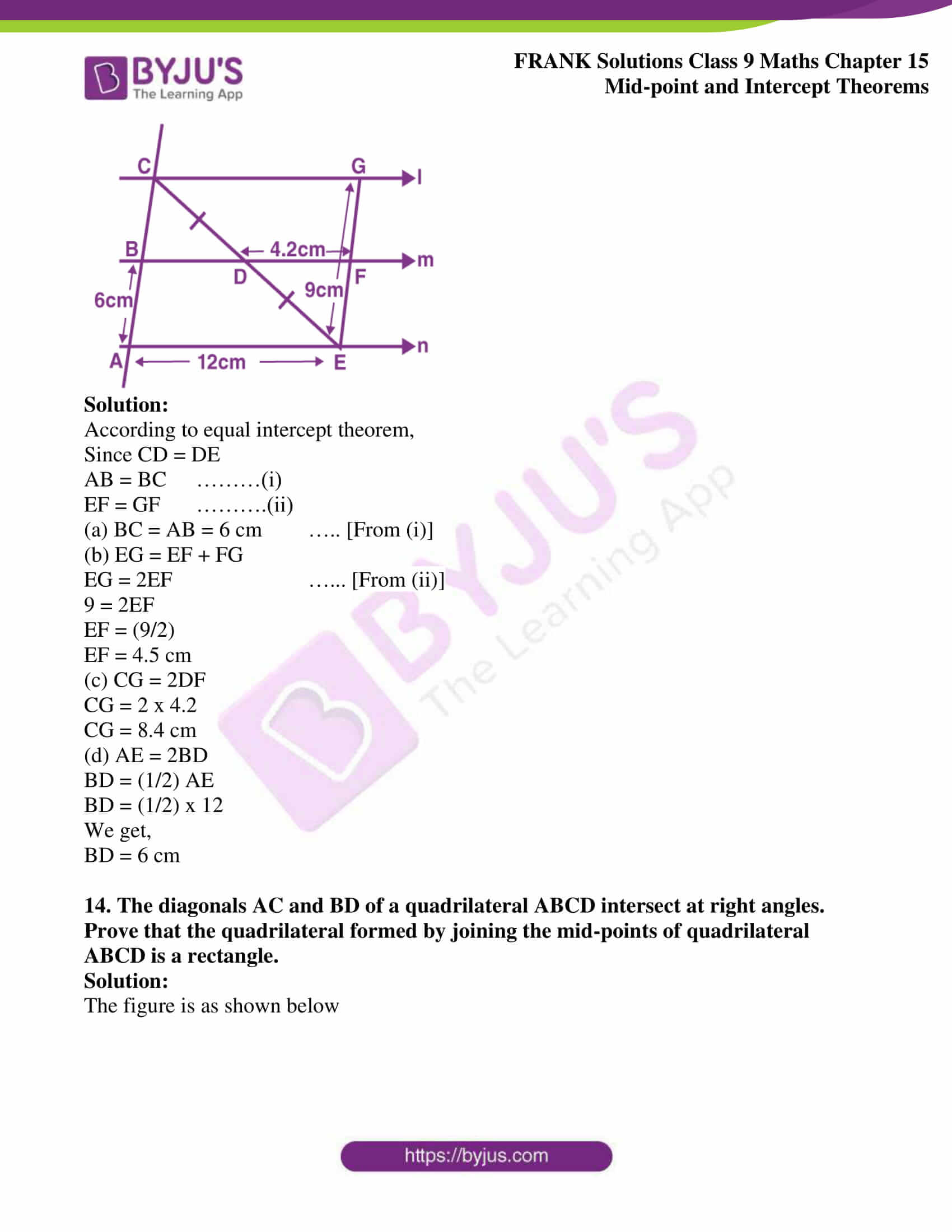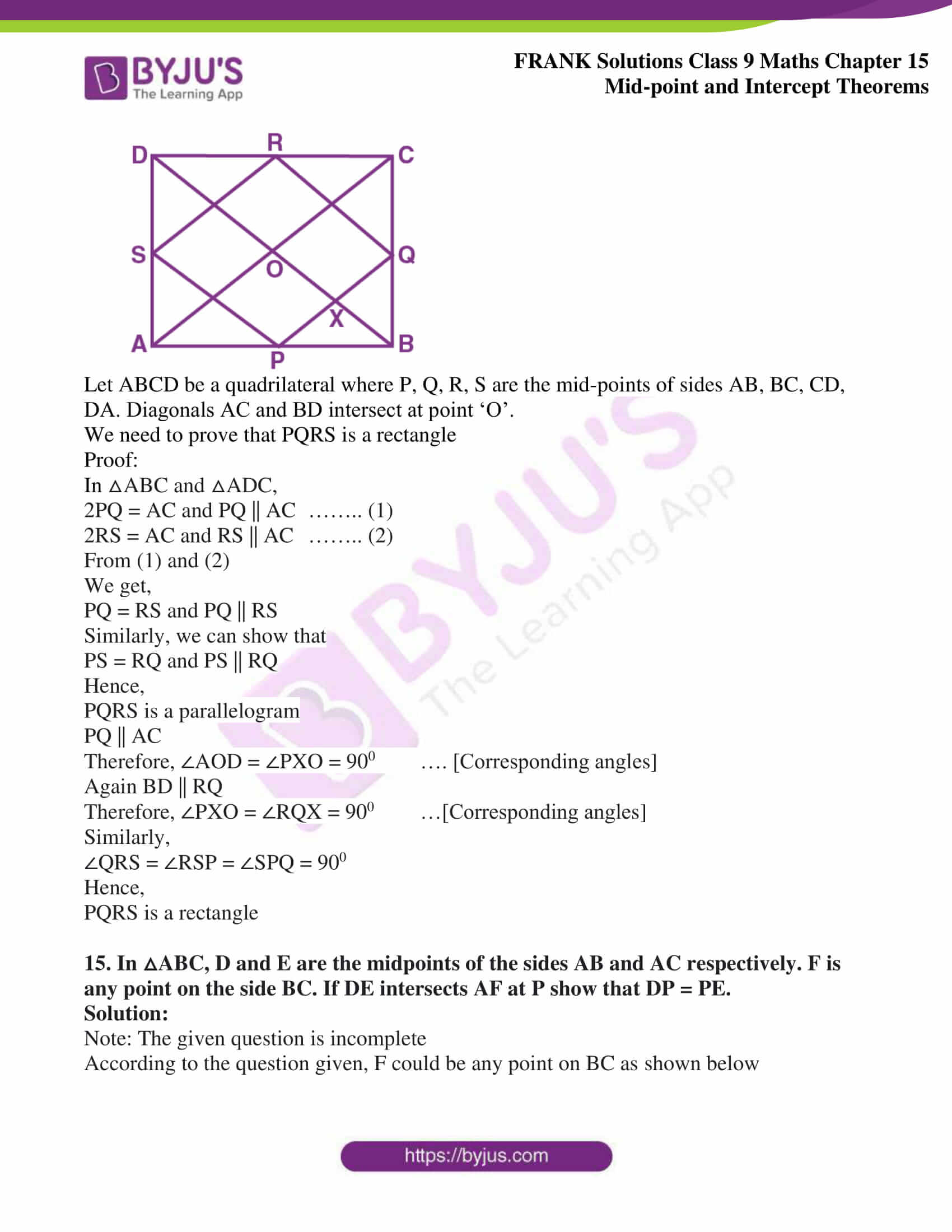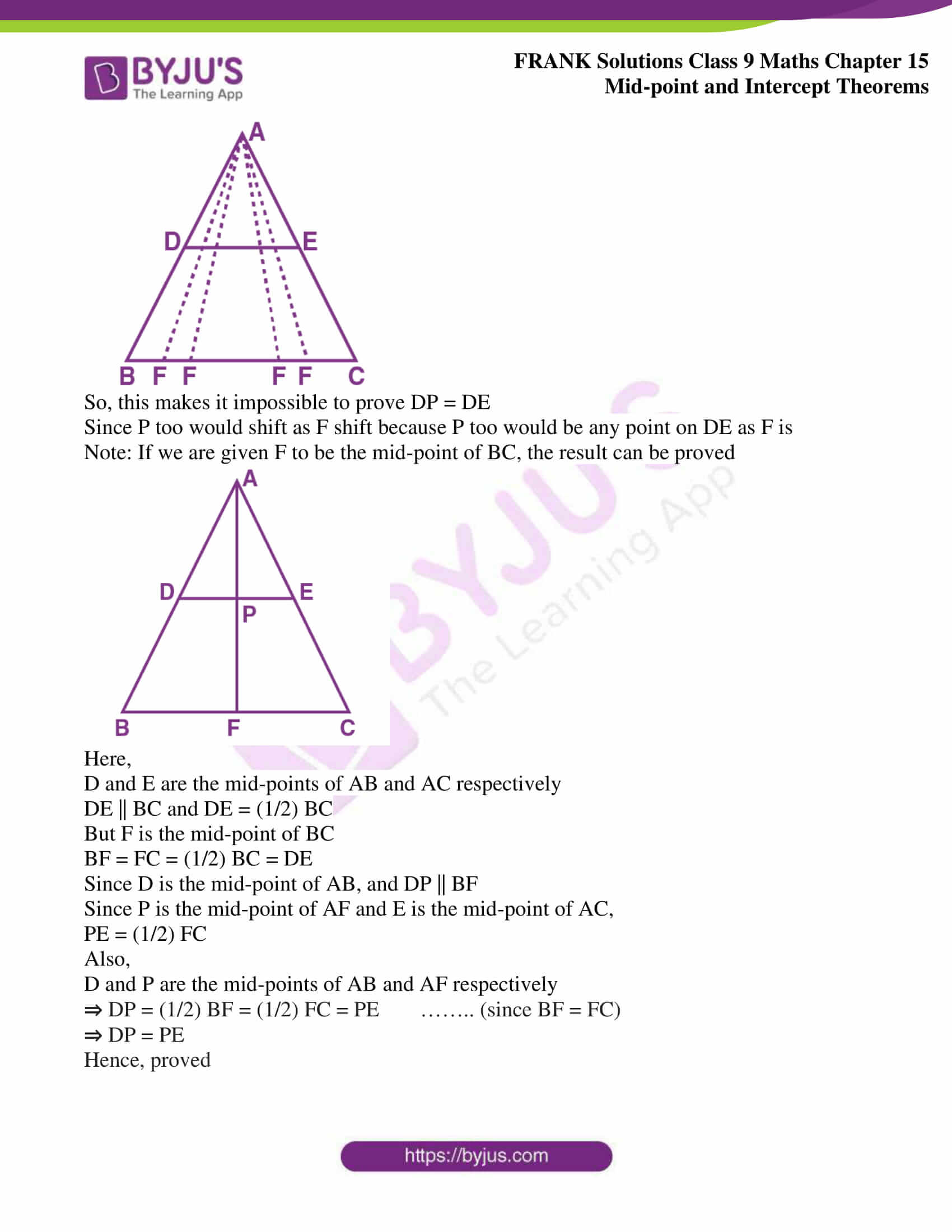## Access Frank Solutions for Class 9 Maths Chapter 15 Mid – point and Intercept Theorems

1. In △ABC, D is the mid-point of AB and E is the mid-point of BC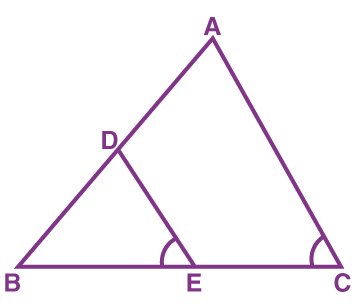Calculate:

(i) DE, if AC = 8.6 cm

(ii) ∠DEB, if ∠ACB = 720

Solution:

In △ABC,

D and E are the mid-points of AB and BC respectively

Hence, by mid-point theorem DE || AC and DE = (1/2) AC

(i) DE = (1/2) AC = (1/2) x 8.6

We get,

= 4.3 cm

(ii) ∠DEB = ∠C = 720 (Corresponding angles are equal, since DE || AC)

2. In △ABC, AB = 12 cm and AC = 9 cm. If M is the mid-point of AB and a straight line through M parallel to AC cuts BC in N, what is the length of MN?

Solution: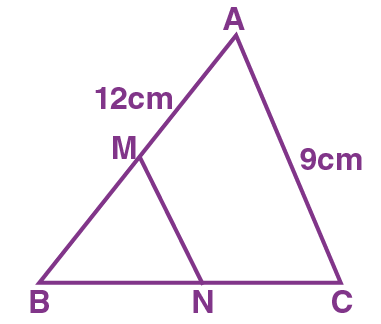MN || AC and M is the mid-point of AB

Hence, N is the mid-point of BC

Therefore, MN = (1/2) AC

= (9/2) cm

We get,

= 4.5 cm

3. (a) In △ABC, D, E, F are the mid-points of BC, CA and AB respectively. Find FE, if BC = 14 cm

(b) In △ABC, D, E, F are the mid-points of BC, CA and AB respectively. Find DE, if AB = 8 cm

(c) In △ABC, D, E, F are the mid-points of BC, CA and AB respectively. Fine ∠FDB if ∠ACB = 1150

Solution:

(a)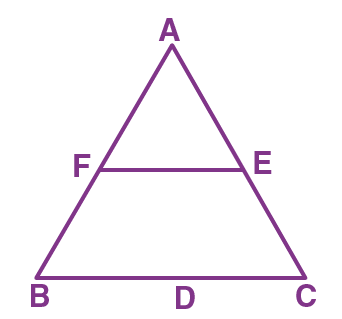F is the mid-point of AB and E is the mid-point of AC

Hence,

FE = (1/2) BC ………… (Mid-point Theorem)

= (1/2) x 14

We get,

= 7 cm

(b)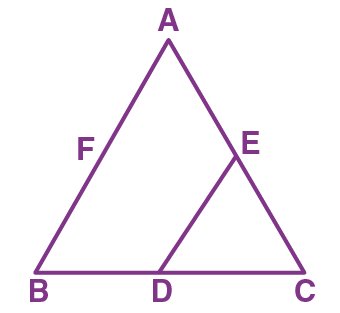In △ABC,

D is the mid-point of BC and E is the mid-point of AC

Hence,

DE = (1/2) AB …….. (Mid-point Theorem)

= (1/2) x 8

We get,

= 4 cm

(c)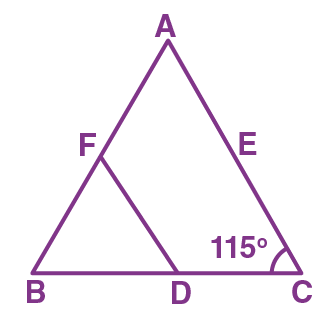In △ABC,

FD || AC

Hence,

∠FDB = ∠ACB = 1150 …….(Corresponding angles are equal)

4. In parallelogram PQRS, L is mid-point of side SR and SN is drawn parallel to LQ which meets RQ produced at N and cuts side PQ at M. Prove that M is the mid-point of PQ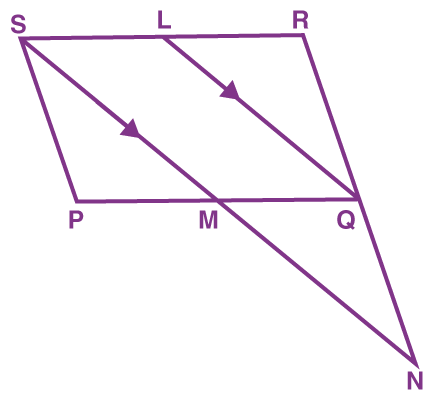Solution:

In △NSR,

MQ = (1/2) SR

But L is the mid-point of SR and SR = PQ (Sides of parallelogram)

So, it can be written as,

MQ = (1/2) PQ

MQ = PM = LS= LR

Hence, M is the mid-point of PQ

5. In △ABC, BE and CF are medians. P is a point on BE produced such that BE = EP and Q is a point on CF produced such that CF = FQ. Prove that:

(a) QAP is a straight line

(b) A is the mid-point of PQ

Solution: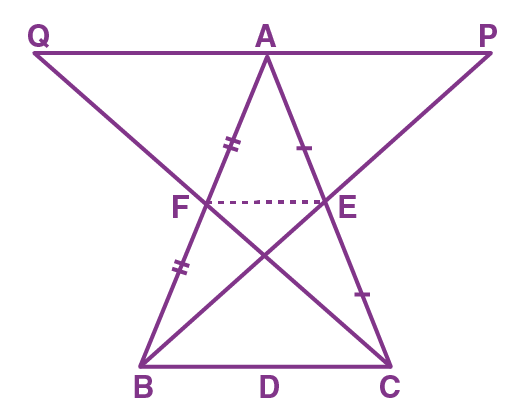Since, BE and CF are the medians,

F is the mid-point of AB and E is the mid-point of AC

We know that the line joining the mid-points of any two sides is parallel and half of the third side

We have,

In △ACQ,

EF|| AQ and EF = (1/2) AQ ………(1)

In △ABP,

EF || AP and EF = (1/2) AP ……….(2)

(a) From (1) and (2)

We get,

AP || AQ (both are parallel to EF)

As AP and AQ are parallel and have a common point A

This is possible only if QAP is a straight line

Thus, proved

(b) From (1) and (2),

EF = (1/2) AQ and EF = (1/2) AP

⇒ (1/2) AQ = (1/2) AP

AQ = AP

Therefore, A is the mid-point of QP

6. Prove that the figure obtained by joining the mid-points of the adjacent sides of a rectangle is a rhombus

Solution: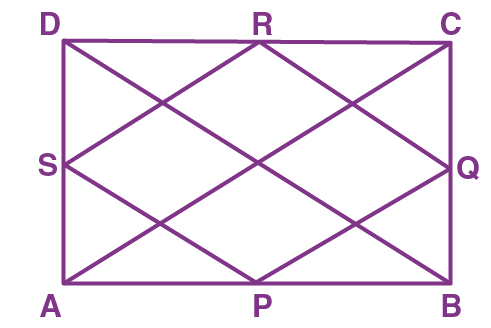In the given rectangle ABCD,

Join AC and BD

In △ABC, P and Q are the mid-points of AB and BC respectively

PQ = (1/2) AC …….. (1) and PQ ||AC

In △BDC, R and Q are the mid-points of CD and BC respectively

QR = (1/2) BD ……..(2) and QR || BD

But AC and BD are diagonals of the rectangle

From equations (1) and (2)

PQ = QR

Similarly,

QR = RS and RS = SP

And,

RS || AC and SP || BD

Therefore, PQ = QR = RS = SP

Hence, PQRS is a rhombus

7. D, E and F are the mid-points of the sides AB, BC and CA of an isosceles △ABC in which AB = BC. Prove that △DEF is also isosceles.

Solution: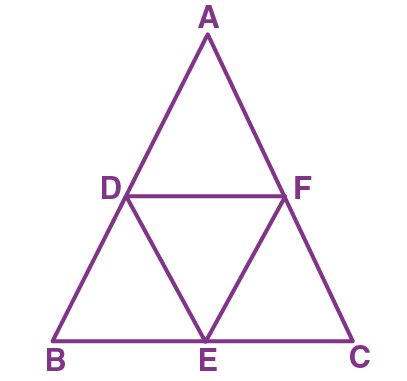E and F are mid-points of BC and AC

Hence, EF = (1/2) AB …….(1)

D and F are the mid-points of AB and AC

Hence, DF = (1/2) BC ……..(2)

But AB = BC

From (1) and (2)

EF = DF

Thus, △DEF is an isosceles triangle

8. The diagonals of a quadrilateral intersect each other at right angle. Prove that the figure obtained by joining the mid-points of the adjacent sides of the quadrilateral is a rectangle.

Solution: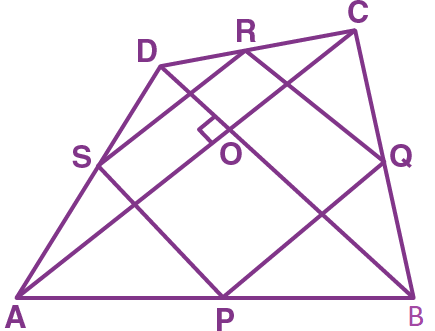P and Q are the mid-points of AB and BC

Hence,

PQ || AC and PQ = (1/2) AC ……. (i)

S and R are the mid-points of AD and DC

Hence,

SR || AC and SR = (1/2) AC …….(ii)

From (i) and (ii),

PQ || SR and PQ = SR

Therefore, PQRS is a parallelogram

Further AC and BC intersect at right angles

∴ SP || BD and BD ⊥ AC

∴ SP ⊥ AC

⇒ SP ⊥ SR

⇒  ∠RSP = 900

∴ ∠RSP = ∠SRQ = ∠RQP = ∠SPQ = 900

Hence, PQRS is a rectangle

9. In a right angled triangle ABC. ∠ABC = 900 and D is the mid-point of AC. Prove that BD = (1/2) AC

Solution: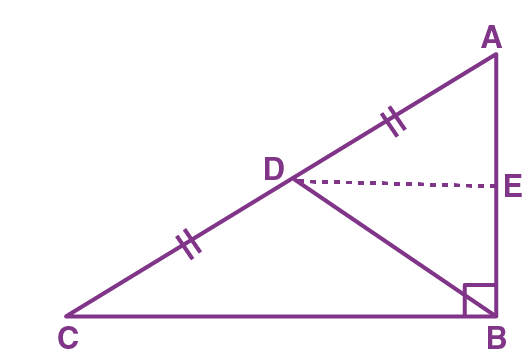Draw line segment DE ||CB, which meets AB at point E

Now,

DE || CB and AB is the transversal,

∴∠AED = ∠ABC …. (corresponding angles)

∠ABC = 900 (given)

⇒ ∠AED = 900

Also,

Since D is the mid-point of AC and DE || CB,

DE bisects side AB,

Hence,

AE = BE …..(i)

In △AED and △BED,

∠AED = ∠BED …..(Each 900)

AE = BE ……[From (i)]

DE = DE ….(Common)

Therefore, △AED △BED ….(By SAS)

⇒ BD = (1/2) AC

Hence, proved

10. In a parallelogram ABCD, E and F are the mid-points of the sides AB and CD respectively. The line segments AF and BF meet the line segments DE and CE at points G and H respectively. Prove that:

(a) △GEA ≅ △GFD

(b) △HEB ≅ △HFC

(c) EGFH is a parallelogram

Solution: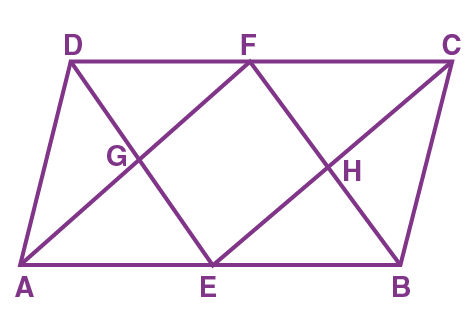Since ABCD is a parallelogram,

AB = CD and AD = BC

Now,

E and F are the mid-points of AB and CD respectively,

Hence,

AE = EB = DF = FC ……….(1)

(a) In △GEA and △GFD,

AE = DF …… [From (1)]

∠AGE = ∠DGF ……… (vertically opposite angles)

∠GAE = ∠GFD …….. (Alternate interior angles)

Therefore,

△GEA ≅ △GFD

(b) In △HEB and △HFC,

BE = FC …..[From (1)]

∠EHB = ∠FHC (vertically opposite angles)

∠HBE = ∠HFC (Alternate interior angles)

Therefore,

△HEB ≅ △HFC

AE = CF …..[From (1)]

AE || CF ……(since AB || DC)

Hence,

AECF is a parallelogram

EC || AF or EH || GF …… (i)

BE = DF ….[From (1)]

BE || DF ….(since AB || DC)

⇒ BEDF is a parallelogram

BF || ED or HF || EG ……(ii)

From equations (i) and (ii),

We get,

EGFH is a parallelogram

11. In △ABC, the medians BE and CD are produced to the points P and Q respectively such that BE = EP and CD = DQ. Prove that:

(a) Q, A and P are collinear

(b) A is the mid-point of PQ

Solution: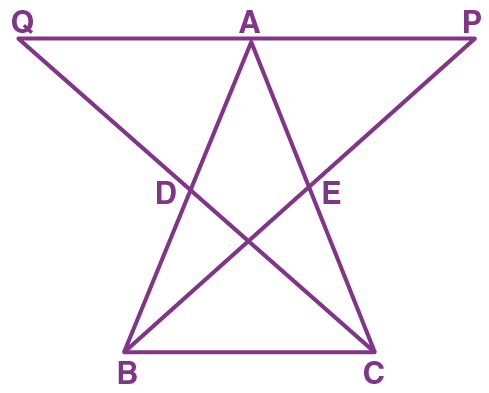CD = DQ … (given)

∠BDC = ∠ADQ ……(vertically opposite angles)

BD = AD ……(D is the mid-point of AB)

Therefore,

∠DBC = ∠DAQ (cpct)…….(i)

And, BC = AQ (cpct) …….(ii)

Similarly,

We can prove

△CEB ≅ △ AEP

∠ECB = ∠EAP (cpct)……..(iii)

And, BC = AP (cpct)……..(iv)

(a) In △ABC,

∠ABC + ∠ACB + ∠BAC = 1800

∠DBC + ∠ECB + ∠BAC = 1800

∠DAQ + ∠EAP + ∠BAC = 1800 [From (i) and (iii)]

Q, A, P are collinear

(b) From (ii) and (iv),

AQ = AP

Therefore, A is the mid-point of PQ

12. In △ABC, D and E are two points on the side AB such that AD = DE = EB. Through D and E, lines are drawn parallel to BC which meet the side AC at points F and G respectively. Through F and G, lines are drawn parallel to AB which meet the side BC at points M and N respectively. Prove that BM = MN = NC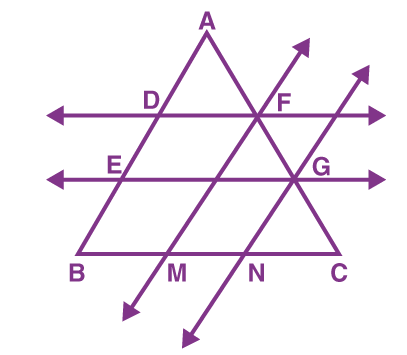Solution:In △AEG,

D is the mid-point of AE and DF || EG

Hence,

F is the mid-point of AG

AF = FG ……….. (1)

In △ABC,

DF || EG|| BC

DE = BE

Hence,

GF = GC …………. (2)

From (1) and (2) we get,

AF = FG = GC

Similarly, since GN|| FM|| AB

Thus, BM = MN = NC (proved)

13. In the given figure, the lines l, m and n are parallel to each other. D is the mid-point of CE. Find

(a) BC

(b) EF

(c) CG

(d) BD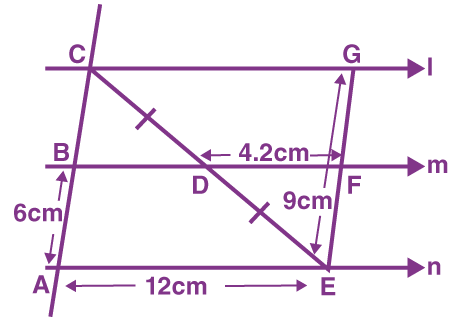Solution:

According to equal intercept theorem,

Since CD = DE

AB = BC ………(i)

EF = GF ……….(ii)

(a) BC = AB = 6 cm ….. [From (i)]

(b) EG = EF + FG

EG = 2EF …… [From (ii)]

9 = 2EF

EF = (9/2)

EF = 4.5 cm

(c) CG = 2DF

CG = 2 x 4.2

CG = 8.4 cm

(d) AE = 2BD

BD = (1/2) AE

BD = (1/2) x 12

We get,

BD = 6 cm

14. The diagonals AC and BD of a quadrilateral ABCD intersect at right angles. Prove that the quadrilateral formed by joining the mid-points of quadrilateral ABCD is a rectangle.

Solution:

The figure is as shown below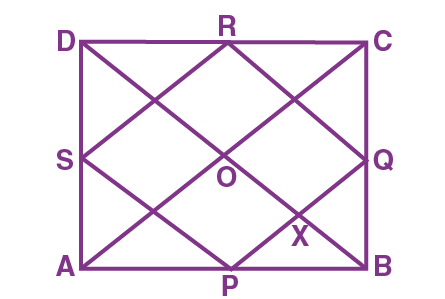Let ABCD be a quadrilateral where P, Q, R, S are the mid-points of sides AB, BC, CD, DA. Diagonals AC and BD intersect at point ‘O’.

We need to prove that PQRS is a rectangle

Proof:

2PQ = AC and PQ || AC …….. (1)

2RS = AC and RS || AC …….. (2)

From (1) and (2)

We get,

PQ = RS and PQ || RS

Similarly, we can show that

PS = RQ and PS || RQ

Hence,

PQRS is a parallelogram

PQ || AC

Therefore, ∠AOD = ∠PXO = 900 …. [Corresponding angles]

Again BD || RQ

Therefore, ∠PXO = ∠RQX = 900 …[Corresponding angles]

Similarly,

∠QRS = ∠RSP = ∠SPQ = 900

Hence,

PQRS is a rectangle

15. In △ABC, D and E are the midpoints of the sides AB and AC respectively. F is any point on the side BC. If DE intersects AF at P show that DP = PE.

Solution:

Note: The given question is incomplete

According to the question given, F could be any point on BC as shown below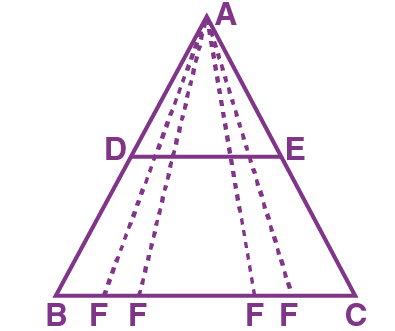So, this makes it impossible to prove DP = DE

Since P too would shift as F shift because P too would be any point on DE as F is

Note: If we are given F to be the mid-point of BC, the result can be proved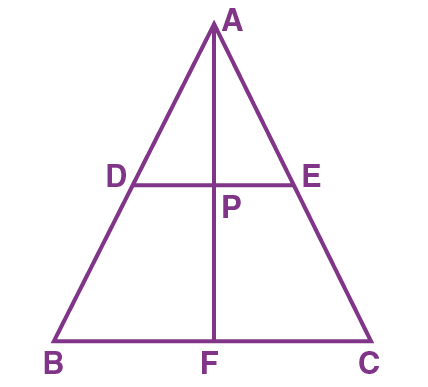Here,

D and E are the mid-points of AB and AC respectively

DE || BC and DE = (1/2) BC

But F is the mid-point of BC

BF = FC = (1/2) BC = DE

Since D is the mid-point of AB, and DP || BF

Since P is the mid-point of AF and E is the mid-point of AC,

PE = (1/2) FC

Also,

D and P are the mid-points of AB and AF respectively

DP = (1/2) BF = (1/2) FC = PE …….. (since BF = FC)

DP = PE

Hence, proved